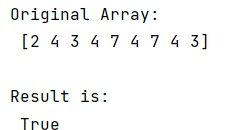# How to determine whether a column/variable is numeric or not in Pandas/NumPy?

Learn, how to determine whether a column/variable is numeric or not in Pandas/NumPy?
Submitted by Pranit Sharma, on December 27, 2022

NumPy is an abbreviated form of Numerical Python. It is used for different types of scientific operations in python. Numpy is a vast library in python which is used for almost every kind of scientific or mathematical operation. It is itself an array which is a collection of various methods and functions for processing the arrays.

## Determining whether a column/variable is numeric or not in Pandas/NumPy

For this purpose, we will use numpy.issubdtype() method to check if the dtype is a sub dtype of a number.

This works for numpy's dtypes but fails for pandas-specific types like pd.Categorical hence if we use the categorical data type we should use is_numeric_dtype() method of pandas.

Let us understand with the help of an example,

## Python code to determine whether a column/variable is numeric or not in Pandas/NumPy

```# Import numpy
import numpy as np

# Creating a numpy array
arr = np.array([2,4,3,4,7,4,7,4,3])

# Display original array
print("Original Array:\n",arr,"\n")

# Determining whether arr is numeric or not
res = np.issubdtype(arr.dtype, np.number)

# Display result
print("Result is:\n",res,"\n")
```

Output: